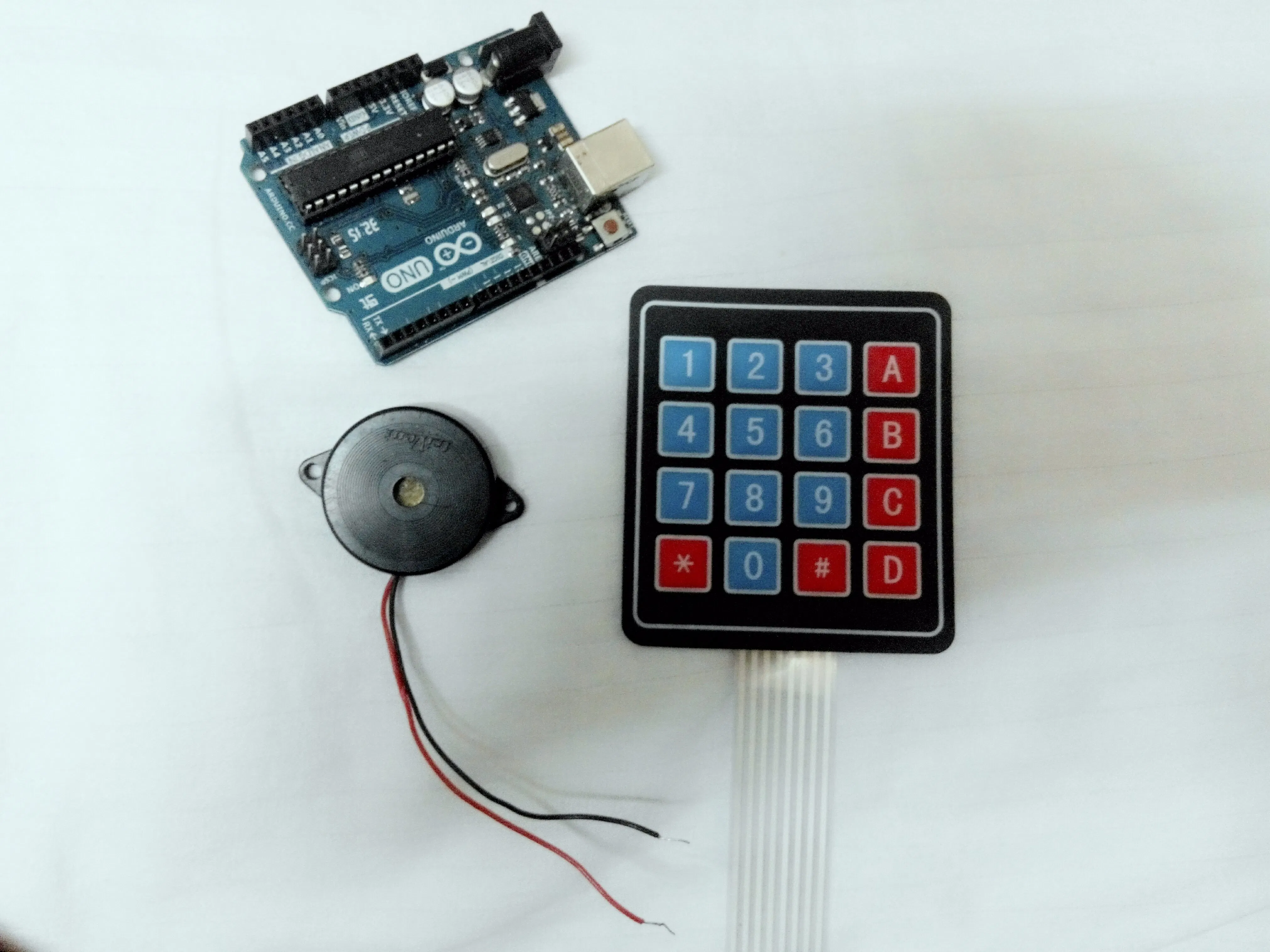Project tutorialArduino as a Piano © GPL3+

Generate the basic notes of a piano using Fast PWM.

• 2,576 views
• 12 respects

Components and suppliesArduino UNO & Genuino UNO
×1
 Piezoelectric buzzer
×1
×1

What this project is about

What this project represents is: you can generate close to any desired frequency using Fast Pulse Width Modulation on any microcontroller up to a top frequency limit (depending on the system clock). Here, Arduino Uno is used, which has 16 MHz system clock. So, you can generate a maximum frequency of roughly 8 MHz using PWM.

The frequencies of basic notes of a piano are as follows:

• c - 261 Hz
• d - 294 Hz
• e - 329 Hz
• f - 349 Hz
• g - 392 Hz
• a - 440 Hz
• b - 493 Hz
• C - 523 Hz

For beginners, this might go over their heads, and for people with non technical background this might be an 'all-greek-to-me' thing. I'm gonna make it simple.

There is an amazing tutorial about the same on Arduino's website. That's where I read and understood it. Basically, what you have to worry about is just 2 registers - TCCR2A and TCCR2B (2 because we are using timer 2 here).

• TCCR2A - Timer/Counter Control Register A for Timer 2)
• TCCR2B - Timer/Counter Control Register B for Timer 2)

These are 8 bit registers and there 8 bits are as follows :

• TCCR2A - [COM2A1, COM2A0, COM2B1, COM2B0, reserved, reserved, WGM21, WGM20]
• TCCR2B - [FOC2A, FOC2B, reserved, reserved, WGM22, CA22, CA21, CS20]

A) Setting WGM22 WGM21 WGM20 as 111 will select the PWM mode - Fast PWM with toggle

B) Setting CA22 CA21 CS20 as 001 will select the pre-scalar as 1 (i.e. the frequency will be divided by 1. For different prescalar values , the table is as follows:

C) Setting COM2A1 COM2A0 as 01 for toggling counter when value reaches top of the OCR2A register. This is the output compare register A. Whatever the value you want your counter to count up to is assigned to this register.

Rest of the bits are 'don't care' for this project.

Generating a frequency:

The formula is: frequency in Hz = 16MHz/prescalar/(OCRA+1)/2 (for register A i.e. pin 11). For e.g. for generating 261 Hz:

• TCCR2A = B01000011; (com2a1 com2a0 bits are 01 for toggling counter when value reaches 119)
• TCCR2B = B00001110; (wgm22 wgm21 wgm20 bits are 111 for fast pwm, ca22 ca21 cs20 bits are 110 for selecting the prescalar 256)
• OCR2A=119;

CALCULATING: 16MHZ/256/120/2 = 260.42 Hz

Similarly, by changing the OCR2A values you can generate rest of the frequencies using this formula. These frequencies are played by a piezoelectric buzzer, which has its +Ve terminal connected to the pin 11 of Arduino Uno and its -Ve terminal to the ground.

Now, for piano keys, I used a 4x4 membrane keypad. For its interfacing refer to my previous project. Use a better keypad and better buzzer for better results. Its code is shown in code1. You can use keypad library instead of this code if you want, to shorten the code. The basic 8 notes are shown in video 1.

I also played the melody of 'Happy birthday to you', but its half (and twice as bad). It is shown in video2.

Code

code 1Arduino
#define c3 9
#define c2 8
#define c1 7
#define c0 6
#define r3 5
#define r2 4
#define r1 3
#define r0 2

void setup() {
Serial.begin(9600);
pinMode(c0,INPUT_PULLUP);
pinMode(c1,INPUT_PULLUP);
pinMode(c2,INPUT_PULLUP);
pinMode(c3,INPUT_PULLUP);
pinMode(r0,OUTPUT);
pinMode(r1,OUTPUT);
pinMode(r2,OUTPUT);
pinMode(r3,OUTPUT);
pinMode(11,OUTPUT);

void loop()
{digitalWrite(r0,LOW);
digitalWrite(r1,HIGH);
digitalWrite(r2,HIGH);
digitalWrite(r3,HIGH);
TCCR2A = B01000011;
TCCR2B = B00001110;
OCR2A=119;
delay(100);
TCCR2B=B00001000;
TCCR2A = B00000000;
digitalWrite(11,LOW);}
TCCR2A = B01000011;
TCCR2B = B00001110;
OCR2A=106;
delay(100);
TCCR2B=B00001000;
TCCR2A = B00000000;
digitalWrite(11,LOW);}
TCCR2A = B01000011;
TCCR2B = B00001110;
OCR2A=94;
delay(100);
TCCR2B=B00001000;
TCCR2A = B00000000;
digitalWrite(11,LOW);}
TCCR2A = B01000011;
TCCR2B = B00001110;
OCR2A=89;
delay(100);
TCCR2B=B00001000;
TCCR2A = B00000000;
digitalWrite(11,LOW);}

digitalWrite(r0,HIGH);
digitalWrite(r1,LOW);
digitalWrite(r2,HIGH);
digitalWrite(r3,HIGH);
TCCR2A = B01000011;
TCCR2B = B00001110;
OCR2A=79;
delay(100);
TCCR2B=B00001000;
TCCR2A = B00000000;
digitalWrite(11,LOW);}
TCCR2A = B01000011;
TCCR2B = B00001110;
OCR2A=71;
delay(100);
TCCR2B=B00001000;
TCCR2A = B00000000;
digitalWrite(11,LOW);}
TCCR2A = B01000011;
TCCR2B = B00001110;
OCR2A=63;
delay(100);
TCCR2B=B00001000;
TCCR2A = B00000000;
digitalWrite(11,LOW);}
TCCR2A = B01000011;
TCCR2B = B00001110;
OCR2A=59;
delay(100);
TCCR2B=B00001000;
TCCR2A = B00000000;
digitalWrite(11,LOW);}

digitalWrite(r0,HIGH);
digitalWrite(r1,HIGH);
digitalWrite(r2,LOW);
digitalWrite(r3,HIGH);
TCCR2A = B01000011;
TCCR2B = B00001110;
OCR2A=25;
delay(100);
TCCR2B=B00001000;
TCCR2A = B00000000;
digitalWrite(11,LOW);}
TCCR2A = B01000011;
TCCR2B = B00001110;
OCR2A=20;
delay(100);
TCCR2B=B00001000;
TCCR2A = B00000000;
digitalWrite(11,LOW);}
TCCR2A = B01000011;
TCCR2B = B00001110;
OCR2A=10;
delay(100);
TCCR2B=B00001000;
TCCR2A = B00000000;
digitalWrite(11,LOW);}
TCCR2A = B01000011;
TCCR2B = B00001110;
OCR2A=50;
delay(100);
TCCR2B=B00001000;
TCCR2A = B00000000;
digitalWrite(11,LOW);}

digitalWrite(r0,HIGH);
digitalWrite(r1,HIGH);
digitalWrite(r2,HIGH);
digitalWrite(r3,LOW);

TCCR2A = B01000011;
TCCR2B = B00001110;
OCR2A=50;
delay(100);
TCCR2B=B00001000;
TCCR2A = B00000000;
digitalWrite(11,LOW);}
TCCR2A = B01000011;
TCCR2B = B00001110;
OCR2A=50;
delay(100);
TCCR2B=B00001000;
TCCR2A = B00000000;
digitalWrite(11,LOW);}
TCCR2A = B01000011;
TCCR2B = B00001110;
OCR2A=50;
delay(100);
TCCR2B=B00001000;
TCCR2A = B00000000;
digitalWrite(11,LOW);}
TCCR2A = B01000011;
TCCR2B = B00001110;
OCR2A=50;
delay(100);
TCCR2B=B00001000;
TCCR2A = B00000000;
digitalWrite(11,LOW);}

Schematics

Playing the basic 8 notes
i5qb88ER3OvI4d4FCG3u.mp4
happy birthday to you
VfWicE86hhRfDbAh7bhM.mp4Electronic Piano Keyboard With Preset Songs

Project tutorial by Lindsay Fox

• 88,347 views
• 176 respects

PIANO

Project tutorial by يمنى السيد ندا

• 3,587 views
• 1 respect

Arduino Tutorial : Mini Piano

Project tutorial by the lonely programmer

• 16,692 views
• 27 respects

Unravel Preset Piano Easy Arduino (Even a Ghoul Can Make It)

Project in progress by ExeCuteLi

• 12,560 views
• 26 respects

Arduino + LEDs + MIDI Keyboard + MuseScore = Piano Tutor

Project tutorial by tcucinotta

• 10,106 views